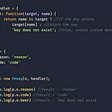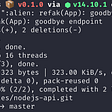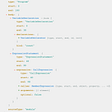# Think like “strings”, not numbers!

`// left shift operator:// how many `0` after `1`?const A = 1 << 0; // 00001const B = 1 << 1; // 00010const C = 1 << 2; // 00100const D = 1 << 3; // 01000const E = 1 << 4; // 10000`

# The AND and the OR

`// & is like boolean &&0 & 0 ✖0 & 1 ✖1 & 0 ✖1 & 1 ✔// | is like boolean ||0 | 0 ✖0 | 1 ✔1 | 0 ✔1 | 1 ✔`
`(A | B)A       00001 |B       00010 =        00011(A | C)A       00001 |C       00100 =        00101(A | B | D)A       00001 |B       00010 |D       01000 =        01011`
`(A | B) & A00011 &00001 =00001 ✔(A | C) & B00101 &00010 =00000 ✖(A | B | D) & D;01011 &01000 =01000 ✔(A | B | D) & C;01011 &00100 =00000 ✖// multiple groups inclusion(A | B | D) & (A | C);01011 &00101 =00001 ✔`
`user.permission = GUEST;if (user.groups.has(developer))  user.permission |= DEVELOPER;`

# The XOR

`// ^ is like a != comparison0 ^ 0 ✖0 ^ 1 ✔1 ^ 0 ✔1 ^ 1 ✖`
`(A | B) ^ A00011 ^00001 =00010 B(A | B | D) ^ D;01011 ^01000 =00011 (A | B)(A | B | D) ^ B;01011 ^00010 =01001 (A | D)// multiple groups removal(A | B | D) ^ (A | D);01011 ^01001 =00010 B`

# ⚠ WARNING

`// C was not in the group before(A | B | D) ^ C;01011 ^00100 =01111 (A | B | C | D)`
• was it there? it’ll go away
• wasn’t it there? it’ll be added
`let toggle = 0;// 0 ^ 1 === 1if ((toggle ^= 1))  console.log('true');// 1 ^ 1 === 0if (!(toggle ^= 1))  console.log('false');// 0 ^ 1 === 1if ((toggle ^= 1))  console.log('true');`

# The all-in case

`const A = 1 << 0; // 00001const B = 1 << 1; // 00010const C = 1 << 2; // 00100const D = 1 << 3; // 01000const E = 1 << 4; // 10000`
`const AtoE = (1 << 5) - 1;// 11111AtoE & A;       // ✔AtoE & B;       // ✔AtoE & (A | C); // ✔const F = 1 << 5;// 100000AtoE & F;       // ✖`

# … and the some-out case …

`AtoE 0000011111FtoJ 1111100000`
`// this one groups them allconst AtoJ = (1 << 10) - 1;// 1111111111// and this one subtract AtoE groupconst FtoJ = AtoJ & ~AtoE;// 1111100000`

# The tilde `~`

• it subtracts `1` to the negative version of the number and return
• it subtracts known `1` from "binary strings" when combined with an AND `&`
`( 0 * -1) - 1;  // -1(-1 * -1) - 1;  //  0`
`// decimal basic example11 & ~1;    // 10// always works as expected with binary strings(parseInt('1111', 2) & ~parseInt('11', 2)).toString(2);// 1100`

# Safer subtracts

`// C was not in the group before(A | B | D) & ~C;// subtract C from (A | B | D) ?01011 &00100 =00000 ✖// B was in the group(A | B | D) & ~B;// subtract B from (A | B | D) ?01011 &00010 =00010 ✔      =01001 (A | D)// multiple subtractions(A | B | D) & ~(A | D);01011 &01001 =01001 ✔      =00010 B// subtracts A only(A | B | D) & ~(A | C);01011 &00101 =00001 ✔      =01010 (B | D)`

# Destructuring a group

`(A | B | D) 01011// find:         A  00001         B  00010         D  01000`
`function* eachValue(group) {  // loop through all multiple of 2 and match  for (let pow = 0, i = 1; i <= group; i = 2 ** ++pow) {    if (group & i)      yield i;  }}// given original A, B, C, D, E constantsfor (const value of eachValue(A | B | D))  console.log(value.toString(2).padStart(5, '0'));// A  00001// B  00010// D  01000`

# Destructuring a subgroup

`AtoE 0000011111FtoJ 1111100000`
`function* eachValue(values, subgroup = -1) {  // remove all undesired `1` from the list of values  // ensure positive number up to (2 ** 32) - 1  const group = (values & subgroup) >>> 0;  // loop through all multiple of 2 and check if these match  for (let pow = 0, i = 1; i <= group; i = 2 ** ++pow) {    if (group & i)      yield i;  }}for (const value of eachValue((A | D | F), AtoE))  console.log(value.toString(2).padStart(5, '0'));// A  00001// D  01000`

# A note about possible optimizations

`function* eachValue(values, filter = ~0) {  let mask = (values & filter) >>> 0, bit = 0;  while (mask) {    if (mask & 1)      yield (1 << bit) >>> 0;    mask >>>= 1;    bit++;  }}`
• the mask is granted to be a positive number up to `Math.pow(2, 32) - 1`
• as long as `mask` is not `0`, the loop keeps going
• if the very first `mask` bit is truthy, or better, just `1`, the value with the related power of `2` is returned, ensuring that if `bit` is exactly `31`, its sign is dropped, so it's always positive.
• the `mask` first right bit is then removed, and the `bit` value is incremented. Please note: as `mask` is granted to be positive, `>>=1` would have likely worked equally well in this case.
`// 0000101001let mask = (A | D | F);//     ↓ ↓  ↓// 0000101001 &// 0000000001 ✔  Aif (mask & 1);// move all 1 one spot on the right ➡mask >>>= 1;//      ↓ ↓  // 0000010100 &// 0000000001 ✖if (mask & 1);mask >>>= 1;//       ↓ ↓ // 0000001010 &// 0000000001 ✖if (mask & 1);mask >>>= 1;//        ↓ ↓// 0000000101 &// 0000000001 ✔  Dif (mask & 1);mask >>>= 1;//         ↓ // 0000000010 &// 0000000001 ✖if (mask & 1);mask >>>= 1;//          ↓// 0000000001 &// 0000000001 ✔  Fif (mask & 1);mask >>>= 1;// 0000000000// end of the loop`

# Other benefits around bitwise operations

• these are extremely fast to compute with every programming language
• every C like programming language handles non-zero integers as truthy, so these are super handy in conditional flows
• there is literally nothing smaller, simpler, or faster when it comes to grouping, and sub-grouping, domain specific values
• it is very difficult to get these wrong, once these are fully grasped, including the XOR operator

# In depth: the left shift operator

`(2 ** 32) - 1;// 11111111111111111111111111111111// as 32bit:  4294967295(2 ** 31) - 1;// 01111111111111111111111111111111// ↑ as 16bit => 2147483647(2 ** 31);// 10000000000000000000000000000000// ↑ as 16bit => -2147483648`
`const i32 = new Int32Array(1);i32 = (2 ** 31) - 1;i32; // 2147483647// increment by 1, reaching 1 << 31i32++;// now it's negativei32; // -2147483648// that is the exact value of 1 << 31i32 === 1 << 31;// true`
`for (let bit = 0; bit < 32; bit++)  console.log(((1 << bit) >>> 0).toString(2).padStart(32, '0'));  // 00000000000000000000000000000001  // to  // 10000000000000000000000000000000`

# Not so limited though …

`// Beyond 32 values: 128 possible values exampleconst big = 0xFFFFFFFFFFFFFFFFFFFFFFFFFFFFFFFFn;big & 0xFn; // truthy`

# Credits

--

--

--

## More from Andrea Giammarchi

Web, Mobile, IoT, and all JS things since 00's. Formerly JS engineer at @nokia, @facebook, @twitter.

Love podcasts or audiobooks? Learn on the go with our new app.

## Develop a Layout Wrapper, One Component for the Entire Website## Understanding Service Providers in Angular## How I built a browser extension with Vue (Part 2)## Introducing Javascript ES6 Proxies## How to Create an Angular Dockerfile## Getters And Setters: What Are Getters And Setters In JavaScript ?## How to Deploy a NodeJS App in 2020## Andrea Giammarchi

Web, Mobile, IoT, and all JS things since 00's. Formerly JS engineer at @nokia, @facebook, @twitter.

## How To Write a Custom ESLint Rule## Rush to Retire NPM for RushJS## Why you should use TypeScript?## WebAssembly : The bridge